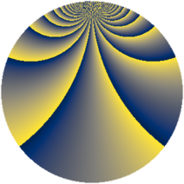# Properties

 Label 1520.2.coLevel $1520$ Weight $2$ Character orbit 1520.co Rep. character $\chi_{1520}(463,\cdot)$ Character field $\Q(\zeta_{12})$ Dimension $240$ Sturm bound $480$

# Related objects

## Defining parameters

 Level: $$N$$ $$=$$ $$1520 = 2^{4} \cdot 5 \cdot 19$$ Weight: $$k$$ $$=$$ $$2$$ Character orbit: $$[\chi]$$ $$=$$ 1520.co (of order $$12$$ and degree $$4$$) Character conductor: $$\operatorname{cond}(\chi)$$ $$=$$ $$380$$ Character field: $$\Q(\zeta_{12})$$ Sturm bound: $$480$$

## Dimensions

The following table gives the dimensions of various subspaces of $$M_{2}(1520, [\chi])$$.

Total New Old
Modular forms 1008 240 768
Cusp forms 912 240 672
Eisenstein series 96 0 96

## Trace form

 $$240q + O(q^{10})$$ $$240q + 24q^{41} + 48q^{57} + 96q^{65} + 48q^{77} + 120q^{81} - 24q^{85} + 96q^{93} + O(q^{100})$$

## Decomposition of $$S_{2}^{\mathrm{new}}(1520, [\chi])$$ into newform subspaces

The newforms in this space have not yet been added to the LMFDB.

## Decomposition of $$S_{2}^{\mathrm{old}}(1520, [\chi])$$ into lower level spaces

$$S_{2}^{\mathrm{old}}(1520, [\chi]) \cong$$ $$S_{2}^{\mathrm{new}}(380, [\chi])$$$$^{\oplus 3}$$